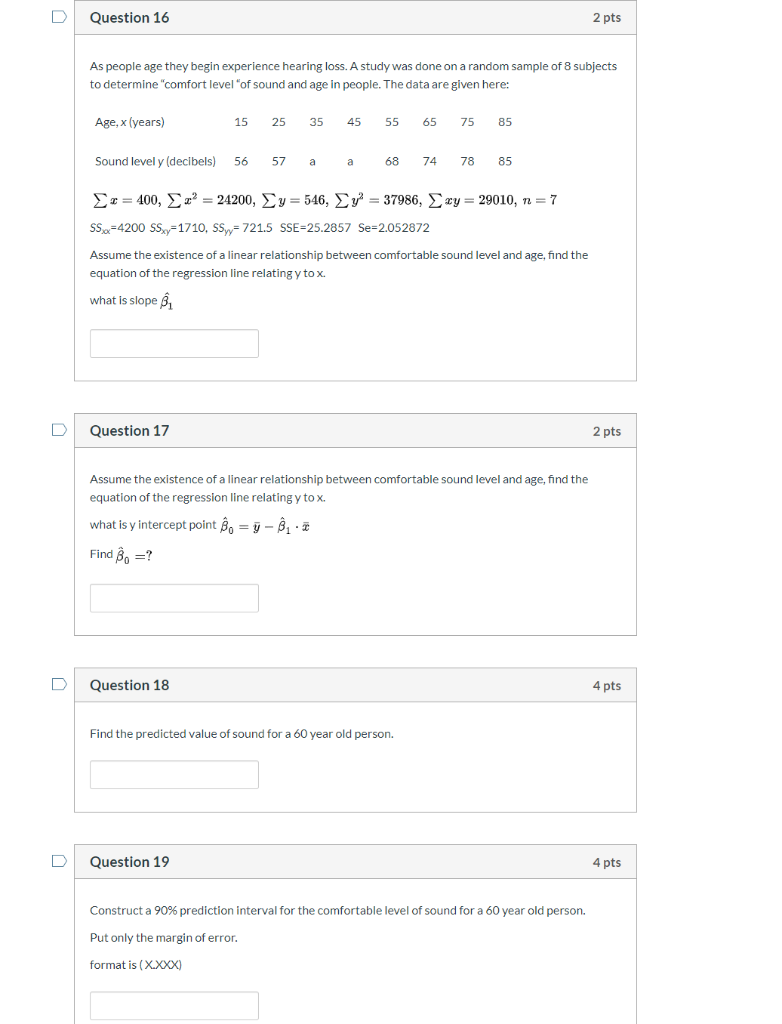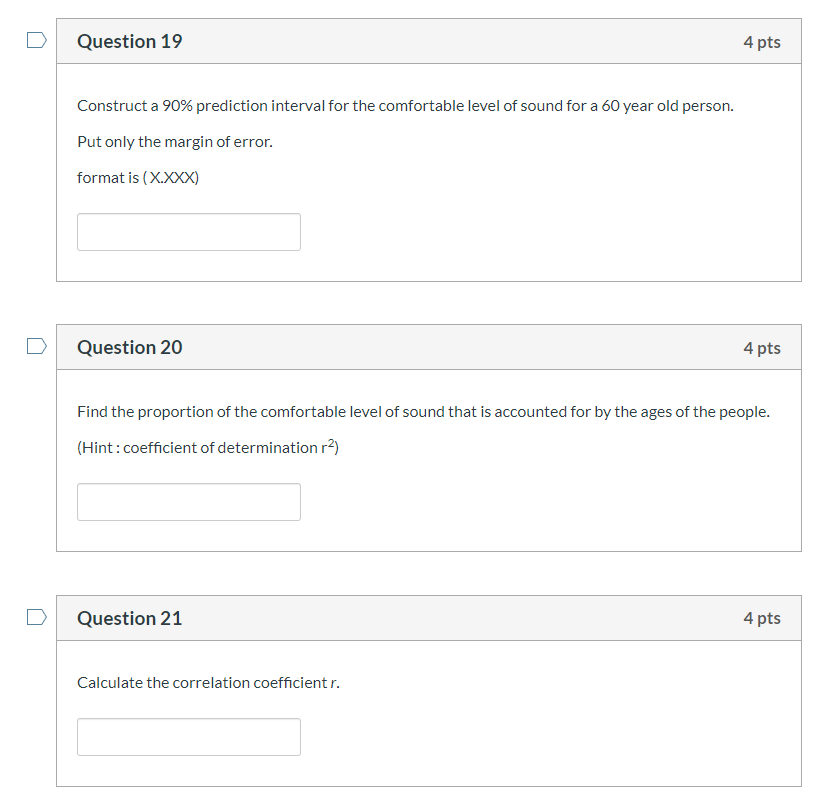# Question 16 2 pts As people age they begin experience hearing loss. A study was done...

###### Question:Question 16 2 pts As people age they begin experience hearing loss. A study was done on a random sample of 8 subjects to determine "comfort level of sound and age in people. The data are given here: Age, x(years) 15 25 35 45 55 65 75 85 Sound level y (decibels) 56 57 a a 68 74 78 85 xy = 29010, n= 7 x = 400, z = 24200, Ey=546, y = 37986, 550=4200 SSxy=1710, SSyy=721.5 SSE=25.2857 Se=2.052872 Assume the existence of a linear relationship between comfortable sound level and age, find the equation of the regression line relating y tox. what is slope B, Question 17 2 pts Assume the existence of a linear relationship between comfortable sound level and age, find the equation of the regression line relating y tox. what is y intercept point B = y - . . * Find =? Question 18 4 pts Find the predicted value of sound for a 60 year old person. Question 19 4 pts Construct a 90% prediction interval for the comfortable level of sound for a 60 year old person. Put only the margin of error. format is (X.XXX)
Question 19 4 pts Construct a 90% prediction interval for the comfortable level of sound for a 60 year old person. Put only the margin of error. format is (X.XXX) Question 20 4 pts Find the proportion of the comfortable level of sound that is accounted for by the ages of the people. (Hint: coefficient of determination r2) Question 21 4 pts Calculate the correlation coefficientr.

#### Similar Solved Questions

##### Listed below are several transactions that took place during the second and third years of operations...
Listed below are several transactions that took place during the second and third years of operations for the RPG Company. Year 2 $260,000 170,000 Year 3$360,000 310,000 Amounts billed to customers for services rendered Cash collected from credit customers Cash disbursements: Payment of rent Salar...
##### What is the amplitude, period and the phase shift of y=2sin3x?
What is the amplitude, period and the phase shift of y=2sin3x?...